【深度学习】深入浅出nnUnet的数据处理方法

## 【深度学习】深入浅出nnUnet的数据处理方法

``````文章目录
1 nnUNet数据预处理crop方法
2 预测结果可视化
3 如何针对三维医学图像分割任务进行通用数据预处理：nnUNet中预处理流程总结
3.1 数据格式转换
3.2 图像裁剪Crop
3.3 重采样Resample
3.4 标准化Normalization
4 后处理``````

# 1 nnUNet数据预处理crop方法

plan_and_preprocess 运行分割，首先需要对数据集进行数据预处理，这也是nnUNet的精髓所在。

``nnUNet_plan_and_preprocess -t NUM --verify_dataset_integrity``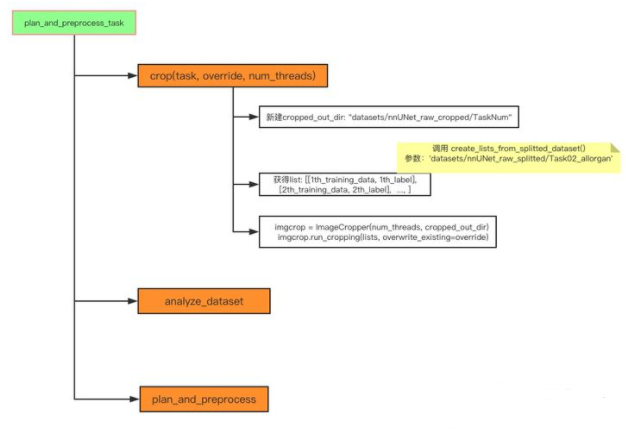``````lists, _ = create_lists_from_splitted_dataset(splitted_4d_output_dir_task)
imgcrop.run_cropping(lists, overwrite_existing=override)

# 2 预测结果可视化

``````import matplotlib.pyplot as plt
import numpy as np
import nrrd
import os
from glob import glob
def plotNrrd(filepath):

x=[]
y=[]
z=[]
for i in range(len(arr0)):
for j in range(len(arr0)):
for k in range(len(arr0)):
if(arr0[i][j][k]==1):
print(i+1,' ',j+1,' ',k+1)
x.append(i+1)
y.append(j+1)
z.append(k+1)
fileindex=(filepath.split('/')[-1]).split('.')

with open('nrrd'+fileindex+'_x.txt','a')as f:
f.write(','.join(map(str,x)))
with open('nrrd'+fileindex+'_y.txt','a')as f1:
f1.write(','.join(map(str,y)))
with open('nrrd'+fileindex+'_z.txt','a')as f2:
f2.write(','.join(map(str,z)))

ax = plt.figure().add_subplot(111, projection = '3d')
#基于ax变量绘制三维图
#xs表示x方向的变量
#ys表示y方向的变量
#zs表示z方向的变量，这三个方向上的变量都可以用list的形式表示
#m表示点的形式，o是圆形的点，^是三角形（marker)
#c表示颜色（color for short）
ax.scatter(x, y, z, c = 'r', marker = '^') #点为红色三角形

#设置坐标轴
ax.set_xlabel('X Label')
ax.set_ylabel('Y Label')
ax.set_zlabel('Z Label')
plt.title(fileindex+'.nrrd')
#显示图像
plt.savefig(filepath+'.png')
if __name__=='__main__':
files = glob('*.nrrd')
for file in files:
plotNrrd(file)
``````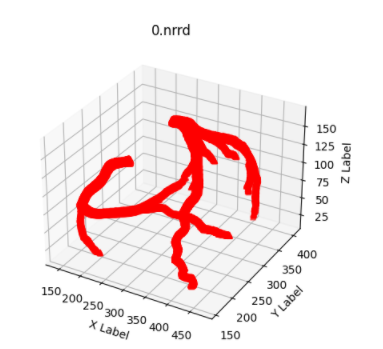# 3 如何针对三维医学图像分割任务进行通用数据预处理：nnUNet中预处理流程总结

## 3.1 数据格式转换

nnUNet中给出了一种建议的目标数据格式，将每一个病例的数据，都存成一个四维numpy数组以及与之对应的pickle文件。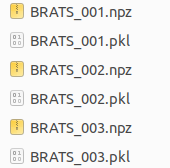MSD Task01_BrainTumor数据转换后存储形式 四维数组array(CXYZ)中，第一个维度的最后array[-1,:,:,:]存储的是分割标注结果。而第一个维度的前面存储不同模态的数据，如MRI数据中有FLAIR, T1w, t1gd, T2w等四种模态，array[0,:,:,:]表示FLAIR序列成像的强度数据，array[1,:,:,:]表示T1加权的强度数据，以此类推。如果仅单模态，则四维数组第一维度长度仅为2，分别表示影像数据以及标注数据。四维数组array的后三个维度代表x,y,z三个坐标表示的三维数据，对于原始影像数据，值大小代表强度，而对于标注结果，后三个维度的三维数据值分别为0，1，2……表示不同的标注类别。在后续的代码中，为了简便，将不同模态的原始图像与分割标注分开，使用data(CXYZ)代表四维图像数据，使用seg(XYZ)代表三维标注数据。

## 3.2 图像裁剪Crop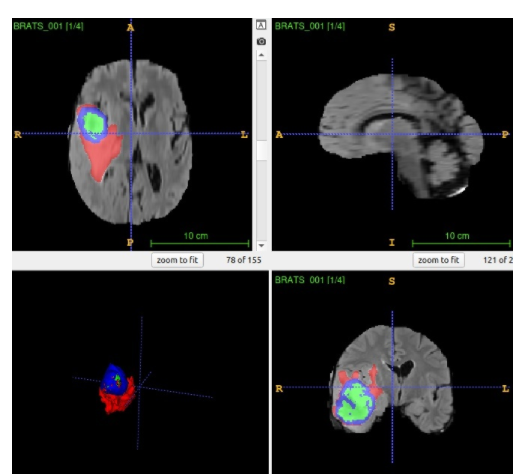大脑MRI影像，外周全0值较多 第一步根据四维图像数据data(C,X,Y,Z)生成三维的非零模板nonzero_mask，标示图像中哪些区域是非零的 。不同的模态都有对应的三维数据，产生不同的三维nonzero_mask，而整个四维图像的非零模板为各个模态非零模板的并集。最后调用scipy库的binary_fill_holes函数对生成的nonzero_mask进行填充。

``````from scipy.ndimage import binary_fill_holes
for c in range(data.shape):

``````mask_voxel_coords = np.where(nonzero_mask != outside_value)
bbox = [[minzidx, maxzidx], [minxidx, maxxidx], [minyidx, maxyidx]]``````

``````resizer = (slice(bbox, bbox), slice(bbox, bbox), slice(bbox, bbox))
cropped_data = []
for c in range(data.shape):
cropped = data[c][resizer]
cropped_data.append(cropped[None])
data = np.vstack(cropped_data)``````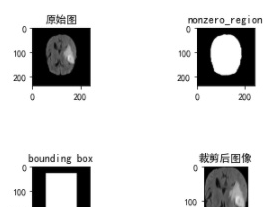为简化，仅展示二维空间中crop的过程图 在对原始数据裁剪完毕之后，使用同样的bounding box对分割标注seg进行裁剪，具体步骤与上述代码的第三步一致。

``````non_zero_label = -1
seg[(seg == 0) & (nonzero_mask == 0)] = nonzero_label``````

## 3.3 重采样Resample

``````anisotropy_threshold = 3

worst_spacing_axis = np.argmax(target)
other_axes = [i for i in range(len(target)) if i != worst_spacing_axis]
other_spacings = [target[i] for i in other_axes]

has_aniso_spacing = target[worst_spacing_axis] > (anisotropy_threshold * min(other_spacings))
has_aniso_voxels = target_size[worst_spacing_axis] * self.anisotropy_threshold < min(other_sizes)
if has_aniso_spacing and has_aniso_voxels:
spacings_of_that_axis = np.vstack(spacings)[:, worst_spacing_axis]
target_spacing_of_that_axis = np.percentile(spacings_of_that_axis, 10)
# don't let the spacing of that axis get higher than the other axes
if target_spacing_of_that_axis < min(other_spacings):
target_spacing_of_that_axis = max(min(other_spacings), target_spacing_of_that_axis) + 1e-5
target[worst_spacing_axis] = target_spacing_of_that_axis``````

``new_shape = np.round(((np.array(original_spacing) / np.array(target_spacing)).astype(float) * shape)).astype(int)``

``````from skimage.transform import resize
if do_separate_z:
if axis == 0:
new_shape_2d = new_shape[1:]
elif axis == 1:
new_shape_2d = new_shape[[0, 2]]
else:
new_shape_2d = new_shape[:-1]
reshaped_final_data = []
for c in range(data.shape):
reshaped_data = []
for slice_id in range(shape[axis]):
if axis == 0:
reshaped_data.append(resize(data[c, slice_id], new_shape_2d, order=3))
elif axis == 1:
reshaped_data.append(resize(data[c, :, slice_id], new_shape_2d, order=3))
else:
reshaped_data.append(resize(data[c, :, :, slice_id], new_shape_2d, order=3))

if shape[axis] != new_shape[axis]:
# The following few lines are blatantly copied and modified from sklearn's resize()
rows, cols, dim = new_shape, new_shape, new_shape
orig_rows, orig_cols, orig_dim = reshaped_data.shape

row_scale = float(orig_rows) / rows
col_scale = float(orig_cols) / cols
dim_scale = float(orig_dim) / dim

map_rows, map_cols, map_dims = np.mgrid[:rows, :cols, :dim]
map_rows = row_scale * (map_rows + 0.5) - 0.5
map_cols = col_scale * (map_cols + 0.5) - 0.5
map_dims = dim_scale * (map_dims + 0.5) - 0.5

coord_map = np.array([map_rows, map_cols, map_dims])
reshaped_final_data.append(map_coordinates(reshaped_data, coord_map, order=0, cval=cval,
mode='nearest')[None])
else:
reshaped = []
for c in range(data.shape):
reshaped.append(resize(data[c], new_shape, order=3)[None])
reshaped_final_data = np.vstack(reshaped)

reshaped_seg = resize(seg, new_shape, order=0)``````

## 3.4 标准化Normalization

nnUNet提供了两种normalization的策略，一种单独针对CT图像，一种应用于其它非CT图像。相同的地方是都使用z-scoring(即减去均值除以标准差)。不同的地方有两点。第一点，CT图像做normalization用的是整个训练集前景的均值和标准差，而非CT图像normalization时仅使用单张图像的灰度信息计算均值和方差。策略不同原因在于，CT图像中，强度信息HU值能反映不同组织的物理性质，用整个训练集前景的统计信息，可以有效的利用HU值的额外信息。第二点，CT图像中经常会有异常大的孤立值和异常小的孤立值，需要先将图像HU值clip到前景HU值[0.5, 99.5]百分比范围之间，而非CT图像没有clip的必要。

``````mask = seg > 0 # 生成前景mask

`````````clike
mean = np.mean(voxels_all)
std = np.std(voxels_all)
percentile_99_5 = np.percentile(voxels_all, 99.5)
percentile_00_5 = np.percentile(voxels_all, 00.5)``````
``````
data[c] = np.clip(data[c], percentile_00_5, percentile_99_5)
data[c] = (data[c] - mean) / std
data[c][seg < 0] = 0 # seg<0代表图像里值为0的背景

```clike
mask = seg >= 0 # 图像中非0区域
else:
data[c] = (data[c] - data[c].mean()) / data[c].std()``````

# 4 后处理

4894
5
95

ember

0个

• 浏览量：3390次
2021-05-24 15:13:24
• 浏览量：2972次
2021-06-23 15:25:25
• 浏览量：3275次
2021-04-27 16:30:07
• 浏览量：4313次
2021-06-09 12:09:57
• 浏览量：3451次
2021-06-22 16:53:40
• 浏览量：3393次
2021-04-20 15:42:26
• 浏览量：3542次
2021-08-09 16:11:19
• 浏览量：3282次
2021-04-20 15:43:03
• 浏览量：3207次
2021-06-16 11:22:18
• 浏览量：3170次
2021-04-21 17:05:28
• 浏览量：3078次
2021-04-21 17:05:56
• 浏览量：3217次
2021-08-02 09:33:43
• 浏览量：5676次
2021-05-12 12:35:30
• 浏览量：4115次
2021-06-15 10:28:29
• 浏览量：8343次
2021-05-04 20:18:49
• 浏览量：3061次
2021-05-17 16:48:29
• 浏览量：5305次
2021-07-08 09:43:47
• 浏览量：9734次
2021-01-08 11:33:04
• 浏览量：2609次
2021-05-18 15:15:50-- 积分
-- EBC